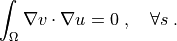# diffusion/laplace_refine_interactive.py¶

Description

Example of solving Laplace’s equation on a block domain refined with level 1 hanging nodes.

The domain is progressively refined towards the edge/face of the block, where Dirichlet boundary conditions are prescribed by an oscillating function.

Findsuch that:## Usage Examples¶

Default options, 2D, storing results in ‘output’ directory:

python3 sfepy/examples/diffusion/laplace_refine_interactive.py output
sfepy-view output/hanging.vtk -2 -f u:wu 1:vw


Default options, 3D, storing results in ‘output’ directory:

python3 sfepy/examples/diffusion/laplace_refine_interactive.py -3 output
sfepy-view output/hanging.vtk -f u:wu:f0.1 1:vw


Finer initial domain, 2D, storing results in ‘output’ directory:

python3 sfepy/examples/diffusion/laplace_refine_interactive.py --shape=11,11 output
sfepy-view output/hanging.vtk -2 -f u:wu 1:vw


Bi-quadratic approximation, 2D, storing results in ‘output’ directory:

python3 sfepy/examples/diffusion/laplace_refine_interactive.py --order=2 output

# View solution with higher order DOFs removed.
sfepy-view output/hanging.vtk -2 -f u:wu 1:vw

# View full solution on a mesh adapted for visualization.
sfepy-view output/hanging_u.vtk -2 -f u:wu 1:vwsource code

#!/usr/bin/env python
r"""
Example of solving Laplace's equation on a block domain refined with level 1
hanging nodes.

The domain is progressively refined towards the edge/face of the block, where
Dirichlet boundary conditions are prescribed by an oscillating function.

Find :math:u such that:

.. math::
\int_{\Omega} \nabla v \cdot \nabla u = 0

Notes
-----
The implementation of the mesh refinement with level 1 hanging nodes is a
proof-of-concept code with many unresolved issues. The main problem is the fact
that a user needs to input the cells to refine at each level, while taking care
of the following constraints:

- the level 1 hanging nodes constraint: a cell that has a less-refined
neighbour cannot be refined;
- the implementation constraint: a cell with a refined neighbour cannot be
refined.

The hanging nodes are treated by a basis transformation/DOF substitution, which
has to be applied explicitly by the user:

- call field.substitute_dofs(subs) before assembling and solving;
- then call field.restore_dofs() before saving results.

Usage Examples
--------------

Default options, 2D, storing results in 'output' directory::

python3 sfepy/examples/diffusion/laplace_refine_interactive.py output
sfepy-view output/hanging.vtk -2 -f u:wu 1:vw

Default options, 3D, storing results in 'output' directory::

python3 sfepy/examples/diffusion/laplace_refine_interactive.py -3 output
sfepy-view output/hanging.vtk -f u:wu:f0.1 1:vw

Finer initial domain, 2D, storing results in 'output' directory::

python3 sfepy/examples/diffusion/laplace_refine_interactive.py --shape=11,11 output
sfepy-view output/hanging.vtk -2 -f u:wu 1:vw

Bi-quadratic approximation, 2D, storing results in 'output' directory::

python3 sfepy/examples/diffusion/laplace_refine_interactive.py --order=2 output

# View solution with higher order DOFs removed.
sfepy-view output/hanging.vtk -2 -f u:wu 1:vw

# View full solution on a mesh adapted for visualization.
sfepy-view output/hanging_u.vtk -2 -f u:wu 1:vw
"""
from __future__ import absolute_import
from argparse import RawDescriptionHelpFormatter, ArgumentParser

import os
import sys
sys.path.append('.')
import numpy as nm

from sfepy.base.base import output, Struct
from sfepy.base.ioutils import ensure_path
from sfepy.mesh.mesh_generators import gen_block_mesh
from sfepy.discrete import (FieldVariable, Integral, Equation, Equations,
Function, Problem)
from sfepy.discrete.fem import FEDomain, Field
from sfepy.discrete.conditions import (Conditions, EssentialBC)
import sfepy.discrete.fem.refine_hanging as rh
from sfepy.solvers.ls import ScipyDirect
from sfepy.solvers.nls import Newton
from sfepy.terms import Term

subs = None
domain = domain0
refine = nm.zeros(domain.mesh.n_el, dtype=nm.uint8)

region = domain.create_region('aux',
'vertices in (%s %.10f)' % (axis, coor),
refine[region.cells] = 1

domain, subs = rh.refine(domain, refine, subs=subs)

return domain, subs

helps = {
'output_dir' :
'output directory',
'dims' :
'dimensions of the block [default: %(default)s]',
'shape' :
'shape (counts of nodes in x, y[, z]) of the block [default: %(default)s]',
'centre' :
'centre of the block [default: %(default)s]',
'3d' :
'generate a 3D block',
'order' :
'field approximation order',
}

def main():
parser = ArgumentParser(description=__doc__.rstrip(),
formatter_class=RawDescriptionHelpFormatter)
action='store', dest='dims',
default='1.0,1.0,1.0', help=helps['dims'])
action='store', dest='shape',
default='7,7,7', help=helps['shape'])
action='store', dest='centre',
default='0.0,0.0,0.0', help=helps['centre'])
action='store_true', dest='is_3d',
default=False, help=helps['3d'])
action='store', dest='order',
default=1, help=helps['order'])
options = parser.parse_args()

dim = 3 if options.is_3d else 2
dims = nm.array(eval(options.dims), dtype=nm.float64)[:dim]
shape = nm.array(eval(options.shape), dtype=nm.int32)[:dim]
centre = nm.array(eval(options.centre), dtype=nm.float64)[:dim]

output('dimensions:', dims)
output('shape:     ', shape)
output('centre:    ', centre)

mesh0 = gen_block_mesh(dims, shape, centre, name='block-fem',
verbose=True)
domain0 = FEDomain('d', mesh0)

bbox = domain0.get_mesh_bounding_box()
min_x, max_x = bbox[:, 0]
eps = 1e-8 * (max_x - min_x)

cnt = (shape - 1) // 2
g0 = 0.5 * dims
grading = nm.array([g0 / 2**ii for ii in range(cnt)]) + eps + centre - g0

domain, subs = refine_towards_facet(domain0, grading, 'x <')

omega = domain.create_region('Omega', 'all')

gamma1 = domain.create_region('Gamma1',
'vertices in (x < %.10f)' % (min_x + eps),
'facet')
gamma2 = domain.create_region('Gamma2',
'vertices in (x > %.10f)' % (max_x - eps),
'facet')

field = Field.from_args('fu', nm.float64, 1, omega,
approx_order=options.order)

if subs is not None:
field.substitute_dofs(subs)

u = FieldVariable('u', 'unknown', field)
v = FieldVariable('v', 'test', field, primary_var_name='u')

integral = Integral('i', order=2*options.order)

t1 = Term.new('dw_laplace(v, u)',
integral, omega, v=v, u=u)
eq = Equation('eq', t1)
eqs = Equations([eq])

def u_fun(ts, coors, bc=None, problem=None):
"""
Define a displacement depending on the y coordinate.
"""
if coors.shape == 2:
min_y, max_y = bbox[:, 1]
y = (coors[:, 1] - min_y) / (max_y - min_y)

val = (max_y - min_y) * nm.cos(3 * nm.pi * y)

else:
min_y, max_y = bbox[:, 1]
min_z, max_z = bbox[:, 2]
y = (coors[:, 1] - min_y) / (max_y - min_y)
z = (coors[:, 2] - min_z) / (max_z - min_z)

val = ((max_y - min_y) * (max_z - min_z)
* nm.cos(3 * nm.pi * y) * (1.0 + 3.0 * (z - 0.5)**2))

return val

bc_fun = Function('u_fun', u_fun)
fix1 = EssentialBC('shift_u', gamma1, {'u.0' : bc_fun})
fix2 = EssentialBC('fix2', gamma2, {'u.all' : 0.0})

ls = ScipyDirect({})

nls = Newton({}, lin_solver=ls)

pb = Problem('heat', equations=eqs)

pb.set_bcs(ebcs=Conditions([fix1, fix2]))

pb.set_solver(nls)

state = pb.solve(save_results=False)

if subs is not None:
field.restore_dofs()

filename = os.path.join(options.output_dir, 'hanging.vtk')
ensure_path(filename)

pb.save_state(filename, state)
if options.order > 1: